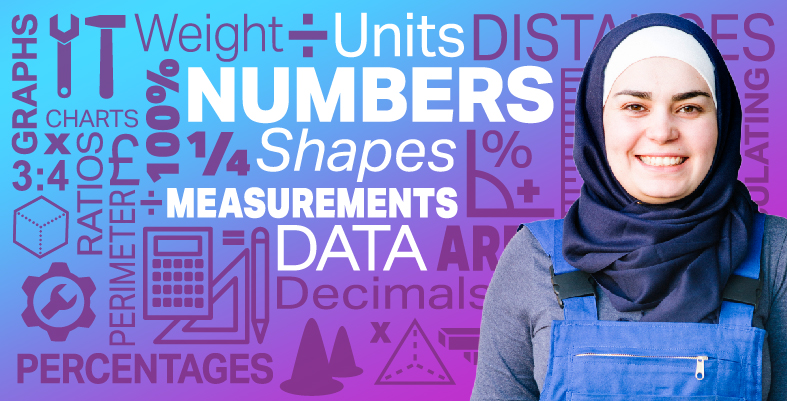Everyday maths for Construction and Engineering 1

Start this free course now. Just create an account and sign in. Enrol and complete the course for a free statement of participation or digital badge if available.

Free course

# 7 Bringing it all together

Congratulations on completing Everyday maths 1. We hope you have enjoyed the experience and now feel inspired to develop your maths skills further.

Throughout this course you have developed your skills within the following areas:

• understanding and using whole numbers, and understanding negative numbers in practical contexts
• adding, subtracting, multiplying and dividing whole numbers using a range of strategies
• understanding and using equivalences between common fractions, decimals and percentages
• adding and subtracting decimals up to two decimal places
• solving simple problems involving ratio, where one number is a multiple of the other
• using simple formulae expressed in words for one- or two-step operations
• solving problems requiring calculation with common measures, including money, time, length, weight, capacity and temperature
• converting units of measure in the same system
• extracting and interpreting information from tables, diagrams, charts and graphs
• collecting and recording discrete data, and organising and representing information in different ways
• finding the mean and range of a group of numbers
• using data to assess the likelihood of an outcome
• identifying various shapes
• working with area and perimeter, scale drawings, and basic map-reading.
FSM_SSC_1